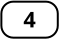Firstly, it is important to indicate on each volumetric flask which standard solution it is intended for (so as to avoid preparing standard solutions with unmarked flasks and thus making mistakes).

Interactive diagram: for each flask, attach the label that corresponds to its standard solutionThen the dilutions required to make the standard solutions for the calibration must be calculated.

Having noted the volumes of stock solution to be taken for each standard solution, you can then proceed to step 3, i.e. the practical task of making the standard solutions of the calibration.

Next page
Previous page